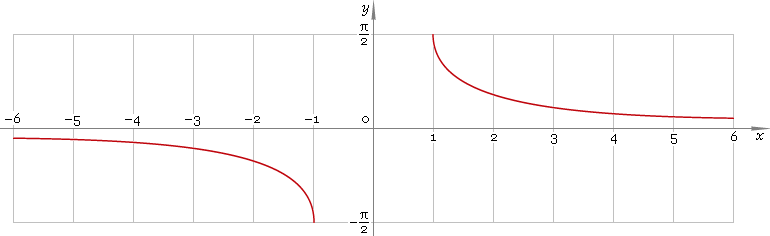The Art of Mathematics

# arccsc or arccosec — trigonometric arc cosecant function

## 1. Definition

Arc cosecant is inverse of the cosecant function.

arccscxcscinvx

## 2. Graph

Arc cosecant is antisymmetric function defined everywhere on real axis, ecxept the range (−1, 1) — so, function domain is (−∞, −1]∪[1, +∞). Its graph is depicted below — fig. 1Fig. 1. Graph of the arc cosecant function y = arccscx.

Function codomain is limited to the range [−π/2, 0)∪(0, π/2].

## 3. Identities

Complementary angle:

arcsecx + arccscx = π/2

and as consequence:

arccsc sec φ = π/2 − φ

Negative argument:

arccsc(−x) = −arccscx

Reciprocal argument:

arcsc(1/x) = arcsinx

Sum and difference:

arccscx + arccscy = arccsc{xy / [x√(1 − 1 /x2) + y√(1 − 1 /y2)]}
arccscx − arccscy = arccsc{xy / [y√(1 − 1 /y2) − x√(1 − 1 /x2)]}

Some argument values:

Argument xValue arccscx
1π/2
√6 − √25π/12
√(50 − 10√5) /52π/5
√(2 − √2)3π/8
2√3 /3π/3
√5 − 13π/10
√2π/4
√(50 + 10√5) /5π/5
2π/6
√(4 + 2√2)π/8
√5 + 1π/10
√6 + √2π/12
Table 1. Arc cosecant for some argument values.

## 4. Derivative and indefinite integral

Arc cosecant derivative:

arccsc′x = −1 /[|x| √(x2 − 1)]

Indefinite integral of the arc cosecant:

∫ arccscx dx = x arccscx + signx ln|x + √(x2 − 1)| + C = x arccscx + arcosh|x| + C

where sign is a signum function and C is an arbitrary constant.

## 5. How to use

To calculate arc cosecant of the number:

``arccsc(−1);``

To get arc cosecant of the complex number:

``arccsc(−1+i);``

To get arc cosecant of the current result:

``arccsc(rslt);``

To get arc cosecant of the number z in calculator memory:

``arccsc(mem[z]);``

## 6. Support

Trigonometric arc cosecant of the real argument is supported in free version of the Librow calculator.

Trigonometric arc cosecant of the complex argument is supported in professional version of the Librow calculator.